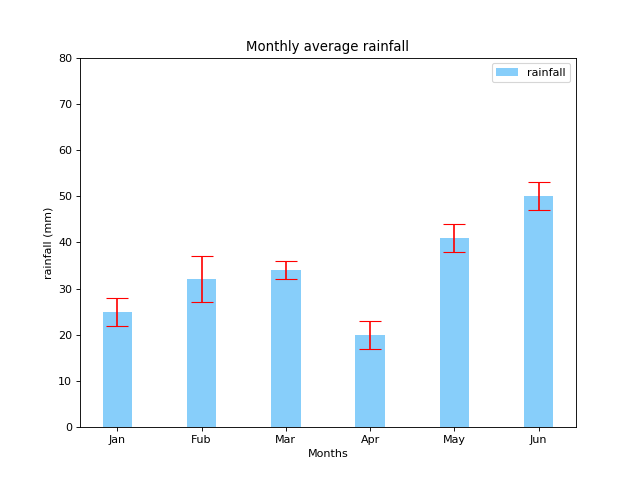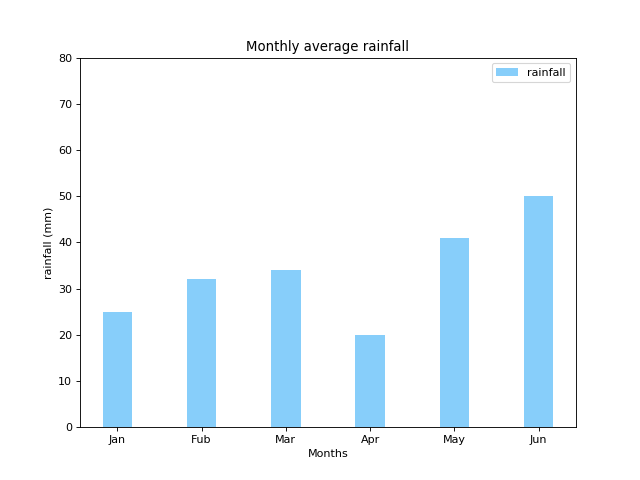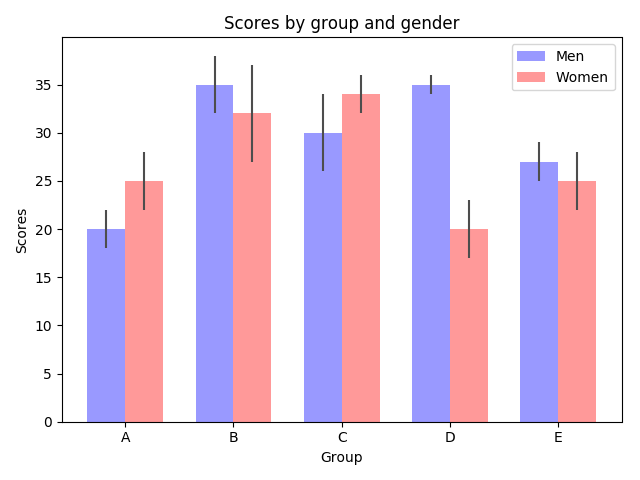• 欢迎访问极客猴，分享 Python 入门，网络爬虫，数据分析，赚钱思维相关的技术与思维`QQ群`
• 本站点文章首发于微信公众号【极客猴】，欢迎关注，第一时间接受文章推送~
• 如果您觉得本站非常有看点，那么赶紧使用 Ctrl+D 收藏极客猴吧

# Python 绘图,我只用 Matplotlib(三)

4年前 (2017-11-23) 168次浏览

## 1.基础

• x

• height

• width

• align

• color

• edgecolor

• linewidth

• tick_label

• xerr

• yerr

• ecolor

• capsize

yerr 参数，那么设置 capsize 参数，会在每跟柱子顶部线段上面的首尾部分增加两条垂直原来线段的线段。对 xerr 参数也是同样道理。可能看说明会觉得绕，如果你看下图就一目了然了。• error_kw

• log

• orientation
设置柱子是显示方式。设置值为 vertical ，那么显示为柱形图。如果设置为 horizontal 条形图。不过 matplotlib 官网不建议直接使用这个来绘制条形图，使用`barh`来绘制条形图。

``````import matplotlib.pyplot as plt
import numpy as np

# 创建一个点数为 8 x 6 的窗口, 并设置分辨率为 80像素/每英寸
plt.figure(figsize=(8, 6), dpi=80)

# 再创建一个规格为 1 x 1 的子图
plt.subplot(1, 1, 1)

# 柱子总数
N = 6
# 包含每个柱子对应值的序列
values = (25, 32, 34, 20, 41, 50)

# 包含每个柱子下标的序列
index = np.arange(N)

# 柱子的宽度
width = 0.35

# 绘制柱状图, 每根柱子的颜色为紫罗兰色
p2 = plt.bar(index, values, width, label="rainfall", color="#87CEFA")

# 设置横轴标签
plt.xlabel('Months')
# 设置纵轴标签
plt.ylabel('rainfall (mm)')

# 添加标题
plt.title('Monthly average rainfall')

# 添加纵横轴的刻度
plt.xticks(index, ('Jan', 'Fub', 'Mar', 'Apr', 'May', 'Jun'))
plt.yticks(np.arange(0, 81, 10))

# 添加图例
plt.legend(loc="upper right")

plt.show()``````## 2.进阶

bar 函数的参数很多，你可以使用这些参数绘制你所需要柱形图的样式。如果你还不会灵活使用这样参数，那就让我们来学习 matplotlib 官方提供的例子。

``````# Credit: Josh Hemann

import numpy as np
import matplotlib.pyplot as plt
from matplotlib.ticker import MaxNLocator
from collections import namedtuple

n_groups = 5

means_men = (20, 35, 30, 35, 27)
std_men = (2, 3, 4, 1, 2)

means_women = (25, 32, 34, 20, 25)
std_women = (3, 5, 2, 3, 3)

fig, ax = plt.subplots()

index = np.arange(n_groups)
bar_width = 0.35

opacity = 0.4
error_config = {'ecolor': '0.3'}

rects1 = ax.bar(index, means_men, bar_width,
alpha=opacity, color='b',
yerr=std_men, error_kw=error_config,
label='Men')

rects2 = ax.bar(index + bar_width, means_women, bar_width,
alpha=opacity, color='r',
yerr=std_women, error_kw=error_config,
label='Women')

ax.set_xlabel('Group')
ax.set_ylabel('Scores')
ax.set_title('Scores by group and gender')
ax.set_xticks(index + bar_width / 2)
ax.set_xticklabels(('A', 'B', 'C', 'D', 'E'))
ax.legend()

fig.tight_layout()
plt.show()``````[赞赏码]